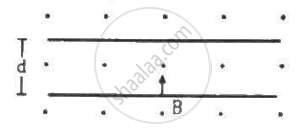Advertisement Remove all ads

# An Electron is Emitted with Negligible Speed from the Negative Plate of a Parallel-plate Capacitor Charged to a Potential Difference V. - Physics

Sum

An electron is emitted with negligible speed from the negative plate of a parallel-plate capacitor charged to a potential difference V. The separation between the plates is dand a magnetic field B exists in the space, as shown in the figure. Show that the electron will fail to strike the upper plates if d > ((2m_eV)/(eB_0^2))^(1/2)Advertisement Remove all ads

#### Solution

Given:-

Potential difference across the plates of the capacitor = V

Separation between the plates = d

Magnetic field intensity = B

The electric field set up between the plates of a capacitor, E = V/d

⇒ a = F/m = (eV)/(M_ed)

⇒ v = sqrt((2eV)/m_e

The electron will move in a circular path due to the given magnetic field. Radius of the circular path,

r = (m_ev)/(eB)

And the electron will fail to strike the upper plate only when the radius of the circular path will be less than d,

i.e d > r

⇒ d > (m_e)/(eB)xx sqrt(2eV)/(m_e)

⇒ d > sqrt(2m_eV)/(eB^2)

Thus, d > ((2m_eV)/(eB_0^2))^{1/2}

Concept: Force on a Moving Charge in Uniform Magnetic and Electric Fields
Is there an error in this question or solution?
Advertisement Remove all ads

#### APPEARS IN

HC Verma Class 11, Class 12 Concepts of Physics Vol. 2
Chapter 12 Magnetic Field
Q 52 | Page 234
Advertisement Remove all ads
Advertisement Remove all ads
Share
Notifications

View all notifications

Forgot password?